# Evaluating Signatures

## Producer Field Guide

HGD_Product
Producer Field Guide
HGD_Portfolio_Suite
Producer

Once signatures are created, they can be evaluated, deleted, renamed, and merged with signatures from other files. Merge signatures to perform complex classifications with signatures that are derived from more than one training method (supervised and/or unsupervised, parametric and/or nonparametric).

Use the Signature Editor to view the contents of each signature, manipulate signatures, and perform your own mathematical tests on the statistics.

Using Signature Data

There are tests to perform that can help determine whether the signature data are a true representation of the pixels to be classified for each class. You can evaluate signatures that were created either from supervised or unsupervised training. The evaluation methods in ERDAS IMAGINE include:

• Alarm—using your own pattern recognition ability, you view the estimated classified area for a signature (using the parallelepiped decision rule) against a display of the original image.
• Ellipse—view ellipse diagrams and scatterplots of data file values for every pair of bands.
• Contingency matrix—do a quick classification of the pixels in a set of training samples to see what percentage of the sample pixels are actually classified as expected. These percentages are presented in a contingency matrix. This method is for supervised training only, for which polygons of the training samples exist.
• Divergence—measure the divergence (statistical distance) between signatures and determine band subsets that maximize the classification.
• Statistics and histograms—analyze statistics and histograms of the signatures to make evaluations and comparisons.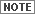If the signature is nonparametric (that is, a feature space signature), you can use only the alarm evaluation method.

After analyzing the signatures, it would be beneficial to merge or delete them, eliminate redundant bands from the data, add new bands of data, or perform any other operations to improve the classification.

Alarm

Use the alarm evaluation to compare an estimated classification of one or more signatures against the original data, as it appears in the View. According to the Parallelepiped decision rule, the pixels that fit the classification criteria are highlighted in the displayed image. You also have the option to indicate an overlap by having it appear in a different color.

With this test, you can use your own pattern recognition skills, or some ground truth data, to determine the accuracy of a signature.

Use Signature Alarm dialog in Signature Editor to perform n-dimensional alarms on the image in the View, using Parallelepiped decision rule. The Signature Alarm dialog creates a functional layer, and in the View you can switch between the image layer and the functional layer.

Ellipse

In this evaluation, ellipses of concentration are calculated with the means and standard deviations stored in the signature file. It is also possible to generate parallelepiped rectangles, means, and labels.

In this evaluation, the mean and the standard deviation of every signature are used to represent the ellipse in 2-dimensional feature space. The ellipse is displayed in a feature space image.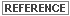Ellipses are explained and illustrated in Feature Space Images under Scatterplots.

When the ellipses in the feature space image show extensive overlap, then the spectral characteristics of the pixels represented by the signatures cannot be distinguished in the two bands that are graphed. In the best case, there is no overlap. Some overlap, however, is expected.

The figure below shows how ellipses are plotted and how they can overlap. The first graph shows how the ellipses are plotted based on the range of 2 standard deviations from the mean. This range can be altered, changing the ellipse plots. Analyzing the plots with differing numbers of standard deviations is useful for determining the limits of a parallelepiped classification.

Ellipse Evaluation of Signatures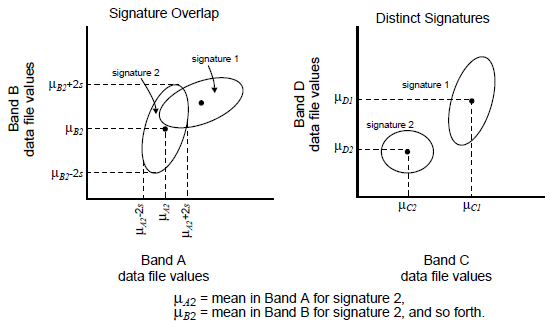By analyzing the ellipse graphs for all band pairs, you can determine which signatures and which bands provide accurate classification results.

Use Signature Editor to create a feature space image and to view ellipses of signature data.

Contingency Matrix

This evaluation classifies all of the pixels in the selected AOIs and compares the results to the pixels of a training sample.

The pixels of each training sample are not always so homogeneous that every pixel in a sample is actually classified to its corresponding class. Each sample pixel only weights the statistics that determine the classes. However, if the signature statistics for each sample are distinct from those of the other samples, then a high percentage of each sample’s pixels is classified as expected.

In this evaluation, a quick classification of the sample pixels is performed using the Minimum Distance, Maximum Likelihood, or Mahalanobis Distance decision rule. Then, a contingency matrix is presented, which contains the number and percentages of pixels that are classified as expected.

Use Signature Editor to perform the contingency matrix evaluation.

Separability

Signature separability is a statistical measure of distance between two signatures. Separability can be calculated for any combination of bands that is used in the classification, thereby ruling out any bands that are not useful in the results of the classification.

For the distance (Euclidean) evaluation, the spectral distance between the mean vectors of each pair of signatures is computed. If the spectral distance between two samples is not significant for any pair of bands, then they may not be distinct enough to produce a successful classification.

The spectral distance is also the basis of the Minimum Distance classification (as explained below). Therefore, computing the distances between signatures helps you predict the results of a Minimum Distance classification.

Use Signature Editor to compute signature separability and distance and automatically generate the report.

The formulas used to calculate separability are related to the Maximum Likelihood decision rule. Therefore, evaluating signature separability helps you predict the results of a Maximum Likelihood classification. The Maximum Likelihood decision rule is explained below.

There are three options for calculating the separability. All of these formulas take into account the covariances of the signatures in the bands being compared, as well as the mean vectors of the signatures.See Math Topics for information on mean vector and covariance matrix.

Divergence

The formula for computing Divergence (Dij) is as follows: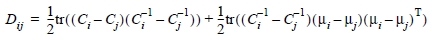Where:

i and j = two signatures (classes) being compared

Ci = covariance matrix of signature i

mi = mean vector of signature i

tr = trace function [see Trace]

T = transposition function [see Transposition]

Source: Swain and Davis, 1978

Transformed Divergence

The formula for computing Transformed Divergence (TD) is as follows: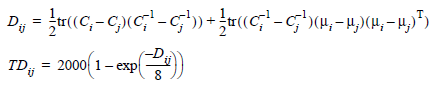Where:

i and j = two signatures (classes) being compared

Ci = covariance matrix of signature i

mi = mean vector of signature i

tr = trace function [see Trace]

T = transposition function [see Transposition]

Source: Swain and Davis, 1978

According to Jensen, the transformed divergence "gives an exponentially decreasing weight to increasing distances between the classes." The scale of the divergence values can range from 0 to 2,000. Interpreting your results after applying transformed divergence requires you to analyze those numerical divergence values. As a general rule, if the result is greater than 1,900, then the classes can be separated. Between 1,700 and 1,900, the separation is fairly good. Below 1,700, the separation is poor (Jensen, 1996).

Jeffries-Matusita Distance

The formula for computing Jeffries-Matusita Distance (JM) is as follows: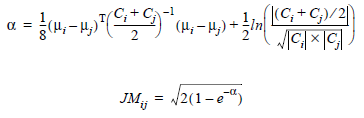Where:

i and j = two signatures (classes) being compared

Ci = covariance matrix of signature i

mi = mean vector of signature i

ln = natural logarithm function

|Ci| = determinant of Ci (matrix algebra)

Source: Swain and Davis, 1978

According to Jensen, "The JM distance has a saturating behavior with increasing class separation like transformed divergence. However, it is not as computationally efficient as transformed divergence" (Jensen, 1996).

Separability Listing

Both transformed divergence and Jeffries-Matusita distance have upper and lower bounds. If the calculated divergence is equal to the appropriate upper bound, then the signatures can be said to be totally separable in the bands being studied. A calculated divergence of zero means that the signatures are inseparable.

• TD is between 0 and 2000.
• JM is between 0 and 1414. That is, the JM values that IMAGINE reports are those resulting from multiplying the values in the formula times 1000.

A separability listing is a report of the computed divergence for every class pair and one band combination. The listing contains every divergence value for the bands studied for every possible pair of signatures.

The separability listing also contains the average divergence and the minimum divergence for the band set. These numbers can be compared to other separability listings (for other band combinations), to determine which set of bands is the most useful for classification.

Weight Factors

As with Bayesian classifier (explained below with Maximum Likelihood), weight factors may be specified for each signature. These weight factors are based on a priori probabilities that any given pixel is assigned to each class. For example, if you know that twice as many pixels should be assigned to Class A as to Class B, then Class A should receive a weight factor that is twice that of Class B.The weight factors do not influence the divergence equations (for TD or JM), but they do influence the report of the best average and best minimum separability.

The weight factors for each signature are used to compute a weighted divergence with the following calculation: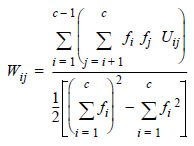Where:

i and j = two signatures (classes) being compared

Uij = unweighted divergence between i and j

Wij = weighted divergence between i and j

c = number of signatures (classes)

fi = weight factor for signature i

Probability of Error

Jeffries-Matusita distance is related to the pairwise probability of error, which is the probability that a pixel assigned to class i is actually in class j. Within a range, this probability can be estimated according to the expression below: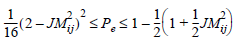Where:

i and j = signatures (classes) being compared

JMij = Jeffries-Matusita distance between i and j

Pe = probability that a pixel is misclassified from i to j

Source: Swain and Davis, 1978

Signature Manipulation

In many cases, training must be repeated several times before the desired signatures are produced. Signatures can be gathered from different sources—different training samples, feature space images, and different clustering programs—all using different techniques. After each signature file is evaluated, you may merge, delete, or create new signatures. The desired signatures can finally be moved to one signature file to be used in the classification.

The following operations upon signatures and signature files are possible with ERDAS IMAGINE:

• View the contents of the signature statistics
• View histograms of the samples or clusters that were used to derive the signatures
• Delete unwanted signatures
• Merge signatures together, so that they form one larger class when classified
• Append signatures from other files. You can combine signatures that are derived from different training methods for use in one classification.

Use Signature Editor to view statistics and histogram listings and to delete, merge, append, and rename signatures within a signature file.